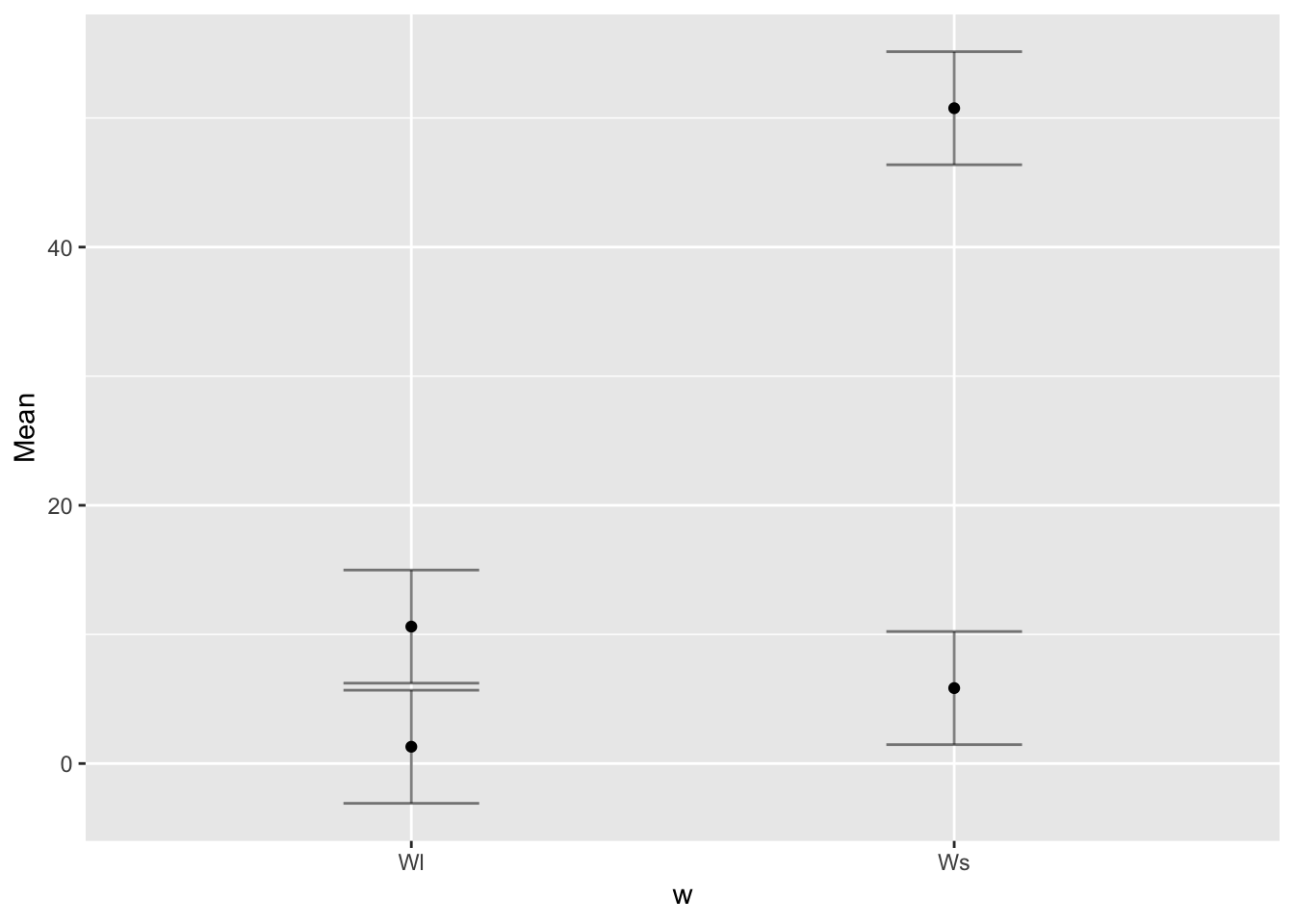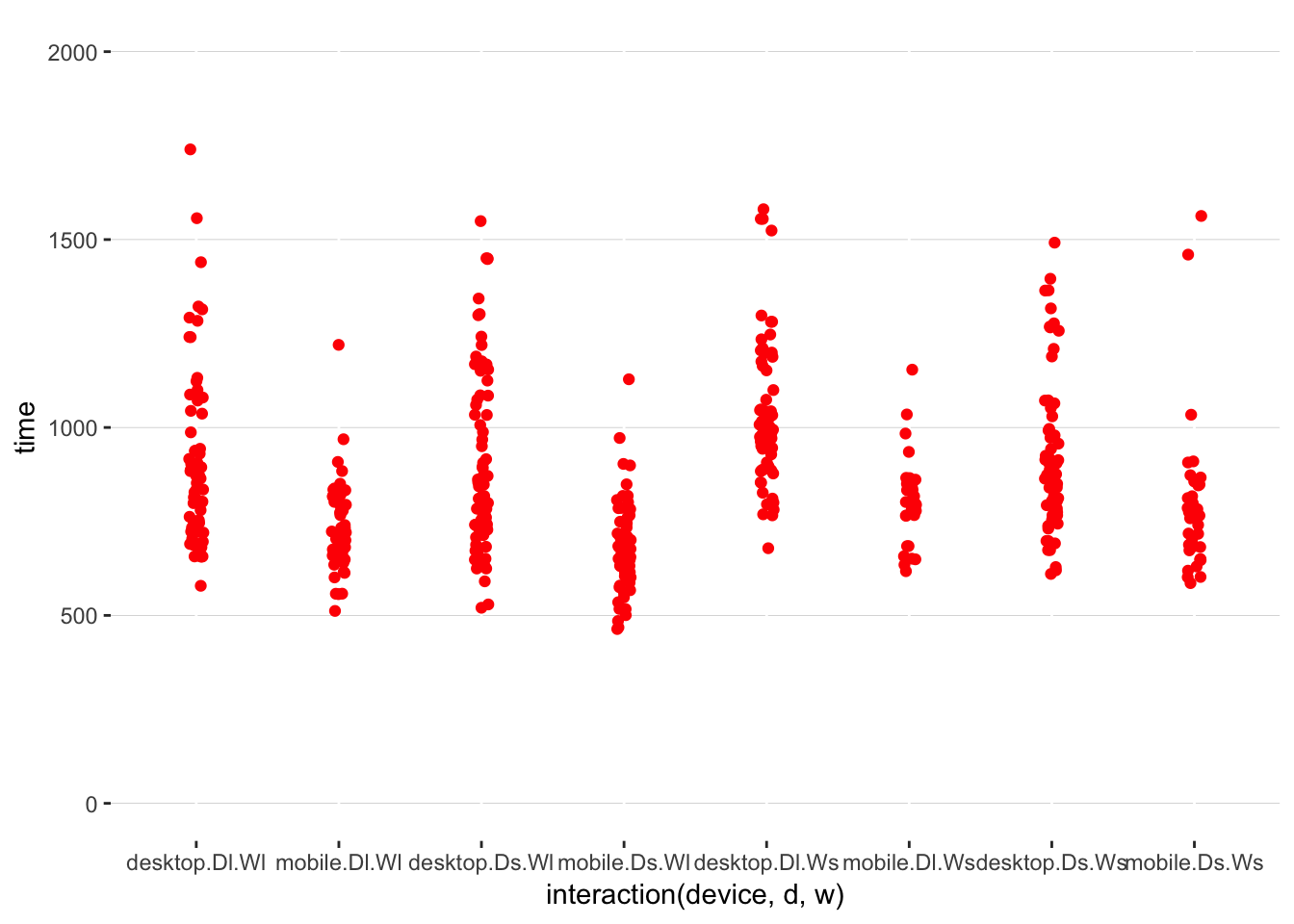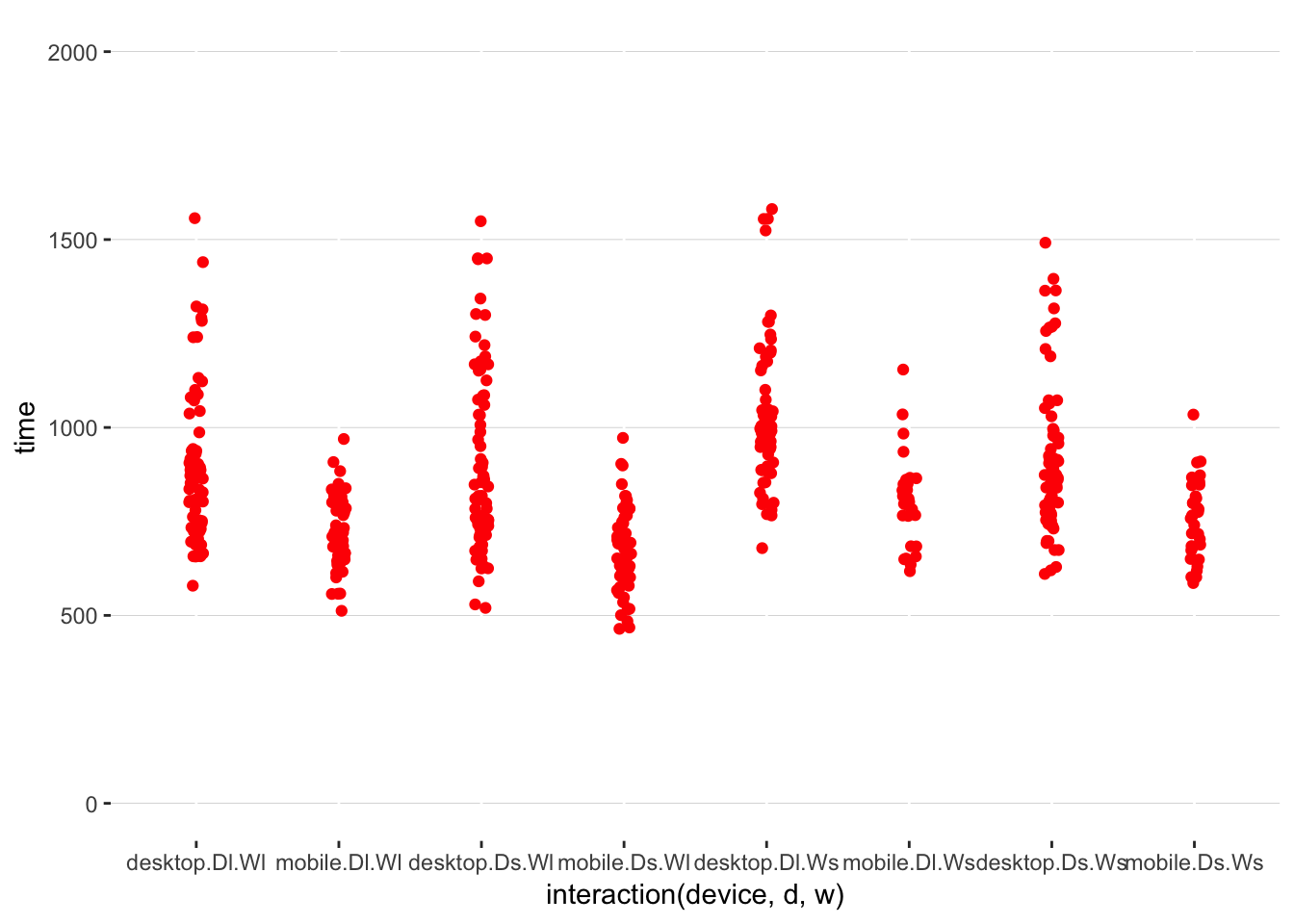# 1 Preparing data

data = read.table("https://cs.uwaterloo.ca/~gcasiez/cs889f18/exercises/fittsdata.csv", header=TRUE, sep=",")
#data = read.table("fittsdata.csv", header=TRUE, sep=",")

## 1.2 Preparing data

We need to add a new column error to indicate 0 or 1 if there is an error as err represents the number of errors made before selecting the target. Directly using err to compute the error rates results in wrong error rates (you can get above 100% in some cases!). We also do some transformations to use more appropriate names.

data_trans =  data %>%
dplyr::rename(device = ismobile) %>% # rename ismobile with more appropriate name
mutate(device = ifelse(device == "True", "mobile", "desktop")) %>% # set proper names
mutate(w = ifelse(w == 0.035, "Ws", "Wl")) %>% # set proper names
mutate(d = ifelse(d == 0.35, "Ds", "Dl")) %>% # set proper names
mutate(error = ifelse(err == 0, 0, 1)) # add a new column error to indicate if there is an error

# 2 Evaluating H1

H1 is “Pointing using indirect interaction (e.g. using a mouse/touchpad) results in lower error rates, especially for small targets”. We need to investigate the effect of device and target size on error rate to answer this question. More interesting, this hypothesis suggests it would exist an interaction between device and target size.

First compute error rate for each participant x device x d x w condition. There is no hypothesis on orientation so we can aggregate the orientations. Also we need some level of aggregation to compute error rates.

data_aggr =   data_trans %>%
group_by(participant, device, d, w) %>%
summarise(errRate = mean(error)*100) 

## 2.1 Run ANOVA on error rate

Convert data using the long format

data.long = melt(data_aggr, id = c("participant","device","d", "w","errRate"))

Indicate what are the independent variables (factors)

data.long$device = factor(data.long$device)
data.long$d = factor(data.long$d)
data.long$w = factor(data.long$w)

We have a mixed design with d and w administrated within subject and device administrated between subject.

results = ezANOVA(data.long, dv=.(errRate), wid=.(participant), within=.(d,w), between=.(device), detailed = TRUE)
kable(results$ANOVA) Effect DFn DFd SSn SSd F p p<.05 ges 1 (Intercept) 1 11 13452.002543 3868.064 38.2548059 0.0000686 * 0.5597425 2 device 1 11 9498.115521 3868.064 27.0107416 0.0002958 * 0.4730468 3 d 1 11 228.862047 4249.803 0.5923763 0.4577113 0.0211726 5 w 1 11 5721.551176 1132.920 55.5529577 0.0000127 * 0.3509717 4 device:d 1 11 190.756214 4249.803 0.4937448 0.4968628 0.0177098 6 device:w 1 11 4095.941937 1132.920 39.7692308 0.0000579 * 0.2790831 7 d:w 1 11 25.429116 1329.693 0.2103646 0.6554112 0.0023976 8 device:d:w 1 11 8.514213 1329.693 0.0704346 0.7956130 0.0008041 kable(results$Mauchly's Test for Sphericity)
kable(results$Sphericity Corrections) kable(anova_apa(results, sph_corr ="gg", print=FALSE)) effect text (Intercept) F(1, 11) = 38.25, p < .001, petasq = .78 device F(1, 11) = 27.01, p < .001, petasq = .71 d F(1, 11) = 0.59, p = .458, petasq = .05 w F(1, 11) = 55.55, p < .001, petasq = .83 device:d F(1, 11) = 0.49, p = .497, petasq = .04 device:w F(1, 11) = 39.77, p < .001, petasq = .78 d:w F(1, 11) = 0.21, p = .655, petasq = .02 device:d:w F(1, 11) = 0.07, p = .796, petasq < .01 kable(ezStats(data.long, dv=.(errRate), wid=.(participant), between=.(device))) ## Coefficient covariances computed by hccm() device N Mean SD FLSD desktop 7 3.571429 6.672321 11.44711 mobile 6 30.681818 11.831286 11.44711 kable(ezStats(data.long, dv=.(errRate), wid=.(participant), within=.(w))) w N Mean SD FLSD Wl 13 5.594406 8.121377 12.61424 Ws 13 26.573427 26.623986 12.61424 kable(ezStats(data.long, dv=.(errRate), wid=.(participant), within=.(w), between=.(device))) device w N Mean SD FLSD desktop Wl 7 1.298701 3.436041 8.761203 desktop Ws 7 5.844156 10.066681 8.761203 mobile Wl 6 10.606061 9.389050 8.761203 mobile Ws 6 50.757576 16.618387 8.761203 ## 2.2 Interpretation of the ANOVA The ANOVA shows a signiciant main effect of device ($$F_{1, 11} = 27.01, p < .001, \eta^2 = .71$$), width ($$F_{1, 11} = 55.55, p < .001, \eta^2 = .83$$) and significant device x width interaction ($$F_{1, 11} = 39.77, p < .001, \eta^2 = .78$$) on error rate. Overall the error rate is 30% with mobile and 3.5% in the desktop condition and increases from 5.6% for the large target to 26.6% for the small one. Let’s analyze where the interaction between device and width comes from. Note that the ANOVA does not provide results for Mauchly’s Test for Sphericity. This is normal as the documentation says “Only reported for effects >2 levels because sphericity necessarily holds for effects with only 2 levels.” Error rates do not necessarily follow a normal distribution, as can be confirmed by the following Shapiro test. check_normal = data_aggr %>% group_by(device, w) %>% summarize(p_value = shapiro.test(errRate)$p)
kable(check_normal)
device w p_value
desktop Wl 0.0000017
desktop Ws 0.0000545
mobile Wl 0.0055549
mobile Ws 0.4626207

## 2.3 Investigating the significant device x width interaction

First investigate using t-test, even we know distributions are not normal.

attach(data.long)
pw <- pairwise.t.test(errRate, interaction(device,w), p.adj = "bonferroni")
detach(data.long)
kable(pw$p.value) desktop.Wl mobile.Wl desktop.Ws mobile.Wl 0.7513503 NA NA desktop.Ws 1.0000000 1e+00 NA mobile.Ws 0.0000000 3e-07 0  ezPlot(data.long, dv=.(errRate), wid=.(participant), within=.(w), between=.(device), x=.(w), do_lines = FALSE)Also investigate using Mann-Whitney pairwise comparisons that are robust to violations of normality. Results show the same significant differences. attach(data.long) pander(pairwise.wilcox.test(errRate, interaction(device,w), p.adj = "bonf")) • method: Wilcoxon rank sum test • data.name: errRate and interaction(device, w) • p.value: desktop.Wl mobile.Wl desktop.Ws mobile.Wl 0.2076 NA NA desktop.Ws 1 1 NA mobile.Ws 4.494e-05 0.001942 0.0002633 • p.adjust.method: bonferroni detach(data.long) kable(aggregate( errRate~device+w, data.long, mean )) device w errRate desktop Wl 1.298701 mobile Wl 10.606061 desktop Ws 5.844156 mobile Ws 50.757576 ## 2.4 Run the analysis using Aligned Rank Transform (ART) ART allows to transform non-normal data to run ANOVA. This was not covered in the previous exercises but this is the best way to analyze the error rate. m <- art(errRate ~ device*d*w + (1|participant), data=data.long) kable(anova(m)) Term F Df Df.res Pr(>F) device device 27.0101715 1 11 0.0002958 d d 3.6782768 1 33 0.0638140 w w 40.3932928 1 33 0.0000003 device:d device:d 0.9121743 1 33 0.3464826 device:w device:w 29.2477257 1 33 0.0000055 d:w d:w 1.2459526 1 33 0.2723890 device:d:w device:d:w 0.0762207 1 33 0.7842075 Results show the same significant effect of device and width and same significant interaction between device and width. ## 2.5 Conclusion Subsequent pairwise comparisons on the significant device x width interaction does not reveal signficiant difference between mobile and desktop for Wl but significant differences between the two device for Ws. As a result we can say that H1 is supported. However these results need to be considered with care as the physical size in millimeters of the targets was not the same between the two devices. The targets were indeed smaller on the mobile screen as their size is defined in percentage of screen width and mobile screens were smaller. Further experiments showing targets with the same physical size on both devices would be required to fully answer this question. However web browers harly allow to determine screens’ pixel densities. # 3 Evaluating H2 H2 is “Pointing times increase with longer distances and smaller target widths”. We need to investigate the effect target distance and size on movement time to answer this question. ## 3.1 Visualizing data We first plot the raw data from the participants data_noerr = data_trans %>% filter(err == 0) ggplot() + geom_jitter(aes(interaction(device,d,w), time), data = data_noerr, colour = I("red"), position = position_jitter(width = 0.05)) + scale_y_continuous(limits = c(0, 2000)) + theme(panel.background = element_blank(), panel.grid.major.y = element_line( size = .1, color = "grey"))We can observe some outliers in the distribution. Maybe some participants got distracted when selecting some targets. We remove the outliers by removing trials 2 standard deviations away from the mean. data_filt = data_trans %>% filter(err == 0) %>% group_by(device,d,w) %>% filter(!(abs(time - mean(time)) > 3*sd(time))) ggplot() + geom_jitter(aes(interaction(device,d,w), time), data = data_filt, colour = I("red"), position = position_jitter(width = 0.05)) + scale_y_continuous(limits = c(0, 2000)) + theme(panel.background = element_blank(), panel.grid.major.y = element_line( size = .1, color = "grey"))We consider only trials with no error and aggregate by participant, device, d and w. data_aggr = data_filt %>% filter(err == 0) %>% group_by(participant, device, d, w) %>% summarise(time = mean(time)) ## 3.2 Run ANOVA on movement time Convert data using the long format data.long = melt(data_aggr, id = c("participant","device","d", "w","time")) Indicate what are the independent variables (factors) data.long$device = factor(data.long$device) data.long$d = factor(data.long$d) data.long$w = factor(data.long$w) results = ezANOVA(data.long, dv=.(time), wid=.(participant), within=.(d,w), between=.(device), detailed = TRUE) kable(results$ANOVA)
Effect DFn DFd SSn SSd F p p<.05 ges
1 (Intercept) 1 11 37225570.511 485826.67 842.8546737 0.0000000 * 0.9799869
2 device 1 11 415283.404 485826.67 9.4027721 0.0107288 * 0.3532828
3 d 1 11 63380.160 111200.75 6.2695777 0.0293019 * 0.0769555
5 w 1 11 96306.091 61204.33 17.3086943 0.0015884 * 0.1124386
4 device:d 1 11 2146.772 111200.75 0.2123591 0.6539018 0.0028159
6 device:w 1 11 3535.460 61204.33 0.6354135 0.4422430 0.0046291
7 d:w 1 11 29074.086 101983.47 3.1359487 0.1042528 0.0368358
8 device:d:w 1 11 3210.703 101983.47 0.3463084 0.5680968 0.0042057
kable(results\$Mauchly&#`